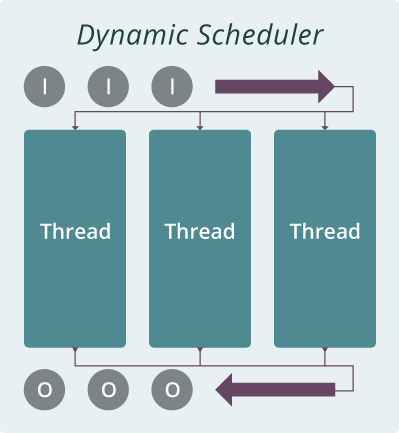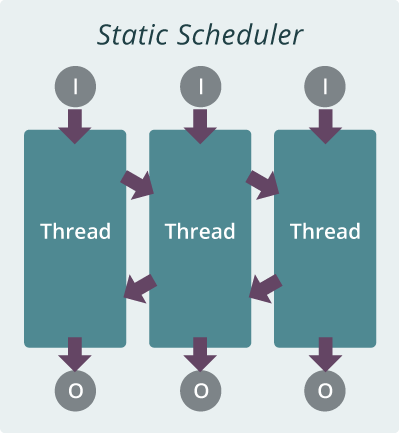dynamic scheduler1. All computation models can use this scheduler.

2. All the inputs are submitted to one queue.

3. Threads dynamically fetch inputs from the queue.

4. The main thread can retrieve the outputs from the output queue

Example

Given three int[] data, find the maximum element in each array. First of all, we need to define the `task`.

```public class FindMaxTask implements Task<int[], Integer> {

@Override
public Integer run(int[] input) throws Exception {
// TODO Auto-generated method stub
int max = Integer.MIN_VALUE;
for(int i=0; i<input.length; i++){
if(max < input[i]){
max = input[i];
}
}
return max;
}
}
```

The `findMaxs` funtion shows how to use dynamic scheduler to run similar tasks in parallel.

```public void findMaxs(){
*/
for (int i = 0; i < numThreads; i++) {
}
/*initialize the dynamic scheduler;
The data type of input is CenPair, the data type of output is Object;
/*Start the Dynamic Scheduler*/
maxCompute.start();

int[] list1 = new int[]{1,2,3,4,5};
int[] list2 = new int[]{14,5,6,7,8,1};
int[] list3 = new int[]{53,43,-1,43,63};
/*Take inputs*/
maxCompute.submit(list1);
maxCompute.submit(list2);
maxCompute.submit(list3);
/*Get results*/
while (maxCompute.hasOutput()) {
Integer out = maxCompute.waitForOutput();
System.out.println(out);
}
}
```

static-scheduler1. All computation models can use this scheduler.

2. Each thread has its own input and output queue.

4. The main thread can retrieve outputs from each task’s output queue.

Example

Given three int[] data, find the maximum element in each array. First of all, we need to define the `task`.

```public class FindMaxTask extends Task<int[], Integer> {

@Override
public Integer run(int[] input) throws Exception {
// TODO Auto-generated method stub
int max = Integer.MIN_VALUE;
for(int i=0; i<input.length; i++){
if(max < input[i]){
max = input[i];
}
}
return max;
}
}
```

The `findMaxs` funtion shows how to use dynamic scheduler to run similar tasks in parallel.

```public void findMaxs(){
/*
*/
for (int i = 0; i < numThreads; i++) {
}
/*
* initialize the dynamic scheduler; The data type of input is CenPair,
* the data type of output is Object; The task is MaxTask
*/
/* Start the Dynamic Scheduler */
maxCompute.start();

int[] list1 = new int[] { 1, 2, 3, 4, 5 };
int[] list2 = new int[] { 14, 5, 6, 7, 8, 1 };
int[] list3 = new int[] { 53, 43, -1, 43, 63 };

/* Specify taskID and take inputs */
maxCompute.submit(1,list1);
maxCompute.submit(2,list2);
maxCompute.submit(3,list3);
/* Get results of task 1*/
while (maxCompute.hasOutput(1)) {
Integer out = maxCompute.waitForOutput(1);
System.out.println(out);
}

/* Get results of task 2*/
while (maxCompute.hasOutput(2)) {
Integer out = maxCompute.waitForOutput(2);
System.out.println(out);
}

/* Get results of task 3*/
while (maxCompute.hasOutput(3)) {
Integer out = maxCompute.waitForOutput(3);
System.out.println(out);
}

}
```